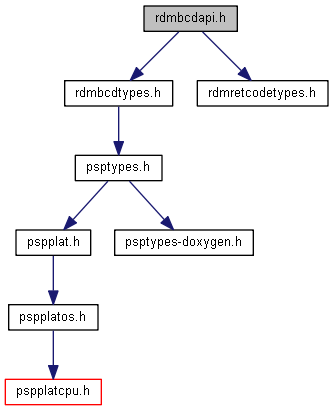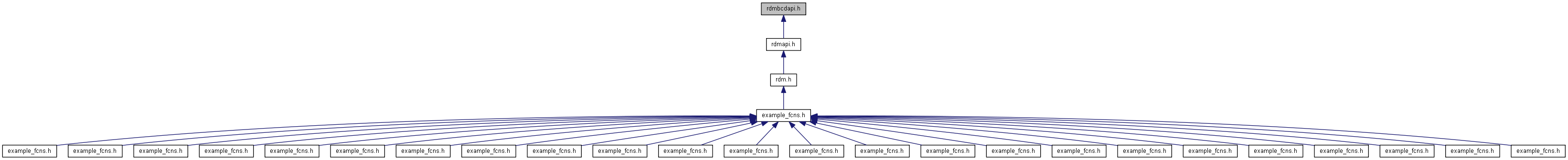rdmbcdapi.h File Reference

Header for Binary-coded decimal (BCD) API. More...

`#include "rdmbcdtypes.h"`
`#include "rdmretcodetypes.h"`
Include dependency graph for rdmbcdapi.h:This graph shows which files directly or indirectly include this file:## Functions

RDM_RETCODE rdm_bcdAdd (const RDM_BCD_T *operand1, const RDM_BCD_T *operand2, RDM_BCD_ROUNDING_MODE rounding_mode, RDM_BCD_T *result)
RDM_RETCODE rdm_bcdAddDouble (const RDM_BCD_T *operand1, double operand2, RDM_BCD_ROUNDING_MODE rounding_mode, RDM_BCD_T *result)
RDM_RETCODE rdm_bcdAddInt32 (const RDM_BCD_T *operand1, int32_t operand2, RDM_BCD_ROUNDING_MODE rounding_mode, RDM_BCD_T *result)
RDM_RETCODE rdm_bcdAddInt64 (const RDM_BCD_T *operand1, int64_t operand2, RDM_BCD_ROUNDING_MODE rounding_mode, RDM_BCD_T *result)
RDM_RETCODE rdm_bcdAddUInt32 (const RDM_BCD_T *operand1, uint32_t operand2, RDM_BCD_ROUNDING_MODE rounding_mode, RDM_BCD_T *result)
RDM_RETCODE rdm_bcdAddUInt64 (const RDM_BCD_T *operand1, uint64_t operand2, RDM_BCD_ROUNDING_MODE rounding_mode, RDM_BCD_T *result)
RDM_RETCODE rdm_bcdAdjust (RDM_BCD_T *argument, uint8_t precision, uint8_t scale)
RDM_RETCODE rdm_bcdCeiling (const RDM_BCD_T *operand, RDM_BCD_T *result)
Take the ceiling of a number. More...
RDM_RETCODE rdm_bcdChgSign (const RDM_BCD_T *operand, RDM_BCD_T *result)
Change the sign of a number. More...
RDM_COMPARE rdm_bcdCompare (const RDM_BCD_T *operand1, const RDM_BCD_T *operand2)
Compare two numbers. More...
void rdm_bcdCopy (const RDM_BCD_T *source, RDM_BCD_T *result)
Copy a BCD number. More...
RDM_RETCODE rdm_bcdCos (const RDM_BCD_T *operand, RDM_BCD_ROUNDING_MODE rounding_mode, RDM_BCD_T *result)
Take the cosine of a number. More...
RDM_RETCODE rdm_bcdCot (const RDM_BCD_T *operand, RDM_BCD_ROUNDING_MODE rounding_mode, RDM_BCD_T *result)
Take the cotangent of a number. More...
RDM_RETCODE rdm_bcdCsc (const RDM_BCD_T *operand, RDM_BCD_ROUNDING_MODE rounding_mode, RDM_BCD_T *result)
Take the cosecant of a number. More...
RDM_RETCODE rdm_bcdDiv (const RDM_BCD_T *operand1, const RDM_BCD_T *operand2, RDM_BCD_ROUNDING_MODE rounding_mode, RDM_BCD_T *result)
Divide two numbers. More...
RDM_RETCODE rdm_bcdDivByDouble (const RDM_BCD_T *operand1, double operand2, RDM_BCD_ROUNDING_MODE rounding_mode, RDM_BCD_T *result)
Divide two numbers. More...
RDM_RETCODE rdm_bcdDivByInt32 (const RDM_BCD_T *operand1, int32_t operand2, RDM_BCD_ROUNDING_MODE rounding_mode, RDM_BCD_T *result)
Divide two numbers. More...
RDM_RETCODE rdm_bcdDivByInt64 (const RDM_BCD_T *operand1, int64_t operand2, RDM_BCD_ROUNDING_MODE rounding_mode, RDM_BCD_T *result)
Divide two numbers. More...
RDM_RETCODE rdm_bcdDivByUInt32 (const RDM_BCD_T *operand1, uint32_t operand2, RDM_BCD_ROUNDING_MODE rounding_mode, RDM_BCD_T *result)
Divide two numbers. More...
RDM_RETCODE rdm_bcdDivByUInt64 (const RDM_BCD_T *operand1, uint64_t operand2, RDM_BCD_ROUNDING_MODE rounding_mode, RDM_BCD_T *result)
Divide two numbers. More...
RDM_RETCODE rdm_bcdDivDouble (double operand1, const RDM_BCD_T *operand2, RDM_BCD_ROUNDING_MODE rounding_mode, RDM_BCD_T *result)
Divide two numbers. More...
RDM_RETCODE rdm_bcdDivInt32 (int32_t operand1, const RDM_BCD_T *operand2, RDM_BCD_ROUNDING_MODE rounding_mode, RDM_BCD_T *result)
Divide two numbers. More...
RDM_RETCODE rdm_bcdDivInt64 (int64_t operand1, const RDM_BCD_T *operand2, RDM_BCD_ROUNDING_MODE rounding_mode, RDM_BCD_T *result)
Divide two numbers. More...
RDM_RETCODE rdm_bcdDivUInt32 (uint32_t operand1, const RDM_BCD_T *operand2, RDM_BCD_ROUNDING_MODE rounding_mode, RDM_BCD_T *result)
Divide two numbers. More...
RDM_RETCODE rdm_bcdDivUInt64 (uint64_t operand1, const RDM_BCD_T *operand2, RDM_BCD_ROUNDING_MODE rounding_mode, RDM_BCD_T *result)
Divide two numbers. More...
RDM_RETCODE rdm_bcdExp (const RDM_BCD_T *operand, RDM_BCD_ROUNDING_MODE rounding_mode, RDM_BCD_T *result)
Take the exponent of a number. More...
RDM_RETCODE rdm_bcdFloor (const RDM_BCD_T *operand, RDM_BCD_T *result)
Take the floor of a number. More...
RDM_RETCODE rdm_bcdFromDouble (double operand, RDM_BCD_T *result)
Convert a double to a BCD number. More...
RDM_RETCODE rdm_bcdFromFloat (float operand, RDM_BCD_T *result)
Convert a float to a BCD number. More...
RDM_RETCODE rdm_bcdFromInt16 (int16_t operand, RDM_BCD_T *result)
Convert an int16_t to a BCD number. More...
RDM_RETCODE rdm_bcdFromInt32 (int32_t operand, RDM_BCD_T *result)
Convert an int32_t to a BCD number. More...
RDM_RETCODE rdm_bcdFromInt64 (int64_t operand, RDM_BCD_T *result)
Convert an int64_t to a BCD number. More...
RDM_RETCODE rdm_bcdFromInt8 (int8_t operand, RDM_BCD_T *result)
Convert an int8_t to a BCD number. More...
RDM_RETCODE rdm_bcdFromString (const char *operand, RDM_BCD_T *result)
Convert a string to a BCD number. More...
RDM_RETCODE rdm_bcdFromUInt16 (uint16_t operand, RDM_BCD_T *result)
Convert an uint16_t to a BCD number. More...
RDM_RETCODE rdm_bcdFromUInt32 (uint32_t operand, RDM_BCD_T *result)
Convert an uint32_t to a BCD number. More...
RDM_RETCODE rdm_bcdFromUInt64 (uint64_t operand, RDM_BCD_T *result)
Convert an uint64_t to a BCD number. More...
RDM_RETCODE rdm_bcdFromUInt8 (uint8_t operand, RDM_BCD_T *result)
Convert an uint8_t to a BCD number. More...
RDM_BOOL_T rdm_bcdIsZero (const RDM_BCD_T *argument)
Is a number zero. More...
RDM_RETCODE rdm_bcdLog (const RDM_BCD_T *operand, RDM_BCD_ROUNDING_MODE rounding_mode, RDM_BCD_T *result)
Take the natual logarithm of a number. More...
RDM_RETCODE rdm_bcdLog10 (const RDM_BCD_T *operand, RDM_BCD_ROUNDING_MODE rounding_mode, RDM_BCD_T *result)
Take the logarithm base 10 of a number. More...
uint8_t rdm_bcdMaxprec (void)
Return the maximum precision. More...
uint8_t rdm_bcdMaxscale (void)
Return the scale. More...
RDM_RETCODE rdm_bcdMult (const RDM_BCD_T *operand1, const RDM_BCD_T *operand2, RDM_BCD_ROUNDING_MODE rounding_mode, RDM_BCD_T *result)
Multiply two numbers. More...
RDM_RETCODE rdm_bcdMultDouble (const RDM_BCD_T *operand1, double operand2, RDM_BCD_ROUNDING_MODE rounding_mode, RDM_BCD_T *result)
Multiply two numbers. More...
RDM_RETCODE rdm_bcdMultInt32 (const RDM_BCD_T *operand1, int32_t operand2, RDM_BCD_ROUNDING_MODE rounding_mode, RDM_BCD_T *result)
Multiply two numbers. More...
RDM_RETCODE rdm_bcdMultInt64 (const RDM_BCD_T *operand1, int64_t operand2, RDM_BCD_ROUNDING_MODE rounding_mode, RDM_BCD_T *result)
Multiply two numbers. More...
RDM_RETCODE rdm_bcdMultUInt32 (const RDM_BCD_T *operand1, uint32_t operand2, RDM_BCD_ROUNDING_MODE rounding_mode, RDM_BCD_T *result)
Multiply two numbers. More...
RDM_RETCODE rdm_bcdMultUInt64 (const RDM_BCD_T *operand1, uint64_t operand2, RDM_BCD_ROUNDING_MODE rounding_mode, RDM_BCD_T *result)
Multiply two numbers. More...
RDM_RETCODE rdm_bcdPi (RDM_BCD_ROUNDING_MODE rounding_mode, RDM_BCD_T *pi)
Pi. More...
RDM_RETCODE rdm_bcdPow (const RDM_BCD_T *operand1, const RDM_BCD_T *operand2, RDM_BCD_ROUNDING_MODE rounding_mode, RDM_BCD_T *result)
Take the power of a number. More...
RDM_RETCODE rdm_bcdPowInt32 (const RDM_BCD_T *operand1, int32_t operand2, RDM_BCD_ROUNDING_MODE rounding_mode, RDM_BCD_T *result)
Take the power of a number. More...
RDM_RETCODE rdm_bcdPowInt64 (const RDM_BCD_T *operand1, int64_t operand2, RDM_BCD_ROUNDING_MODE rounding_mode, RDM_BCD_T *result)
Take the power of a number. More...
RDM_RETCODE rdm_bcdPowUInt32 (const RDM_BCD_T *operand1, uint32_t operand2, RDM_BCD_ROUNDING_MODE rounding_mode, RDM_BCD_T *result)
Take the power of a number. More...
RDM_RETCODE rdm_bcdPowUInt64 (const RDM_BCD_T *operand1, uint64_t operand2, RDM_BCD_ROUNDING_MODE rounding_mode, RDM_BCD_T *result)
Take the power of a number. More...
RDM_RETCODE rdm_bcdReciprocal (const RDM_BCD_T *operand, RDM_BCD_ROUNDING_MODE rounding_mode, RDM_BCD_T *result)
Take the reciprocal of a number. More...
RDM_RETCODE rdm_bcdRound (const RDM_BCD_T *operand, RDM_BCD_T *result)
Find the nearest integer of a BCD number. More...
RDM_RETCODE rdm_bcdSec (const RDM_BCD_T *operand, RDM_BCD_ROUNDING_MODE rounding_mode, RDM_BCD_T *result)
Take the secant of a number. More...
int16_t rdm_bcdSign (const RDM_BCD_T *argument)
Get the sign of a number. More...
RDM_RETCODE rdm_bcdSin (const RDM_BCD_T *operand, RDM_BCD_ROUNDING_MODE rounding_mode, RDM_BCD_T *result)
Take the sine of a number. More...
RDM_RETCODE rdm_bcdSqrt (const RDM_BCD_T *operand, RDM_BCD_ROUNDING_MODE rounding_mode, RDM_BCD_T *result)
Take the square root of a number. More...
RDM_RETCODE rdm_bcdSub (const RDM_BCD_T *operand1, const RDM_BCD_T *operand2, RDM_BCD_ROUNDING_MODE rounding_mode, RDM_BCD_T *result)
Subtract two numbers. More...
RDM_RETCODE rdm_bcdSubDouble (const RDM_BCD_T *operand1, double operand2, RDM_BCD_ROUNDING_MODE rounding_mode, RDM_BCD_T *result)
Subtract two numbers. More...
RDM_RETCODE rdm_bcdSubFromDouble (double operand1, const RDM_BCD_T *operand2, RDM_BCD_ROUNDING_MODE rounding_mode, RDM_BCD_T *result)
Subtract two numbers. More...
RDM_RETCODE rdm_bcdSubFromInt32 (int32_t operand1, const RDM_BCD_T *operand2, RDM_BCD_ROUNDING_MODE rounding_mode, RDM_BCD_T *result)
Subtract two numbers. More...
RDM_RETCODE rdm_bcdSubFromInt64 (int64_t operand1, const RDM_BCD_T *operand2, RDM_BCD_ROUNDING_MODE rounding_mode, RDM_BCD_T *result)
Subtract two numbers. More...
RDM_RETCODE rdm_bcdSubFromUInt32 (uint32_t operand1, const RDM_BCD_T *operand2, RDM_BCD_ROUNDING_MODE rounding_mode, RDM_BCD_T *result)
Subtract two numbers. More...
RDM_RETCODE rdm_bcdSubFromUInt64 (uint64_t operand1, const RDM_BCD_T *operand2, RDM_BCD_ROUNDING_MODE rounding_mode, RDM_BCD_T *result)
Subtract two numbers. More...
RDM_RETCODE rdm_bcdSubInt32 (const RDM_BCD_T *operand1, int32_t operand2, RDM_BCD_ROUNDING_MODE rounding_mode, RDM_BCD_T *result)
Subtract two numbers. More...
RDM_RETCODE rdm_bcdSubInt64 (const RDM_BCD_T *operand1, int64_t operand2, RDM_BCD_ROUNDING_MODE rounding_mode, RDM_BCD_T *result)
Subtract two numbers. More...
RDM_RETCODE rdm_bcdSubUInt32 (const RDM_BCD_T *operand1, uint32_t operand2, RDM_BCD_ROUNDING_MODE rounding_mode, RDM_BCD_T *result)
Subtract two numbers. More...
RDM_RETCODE rdm_bcdSubUInt64 (const RDM_BCD_T *operand1, uint64_t operand2, RDM_BCD_ROUNDING_MODE rounding_mode, RDM_BCD_T *result)
Subtract two numbers. More...
RDM_RETCODE rdm_bcdTan (const RDM_BCD_T *operand, RDM_BCD_ROUNDING_MODE rounding_mode, RDM_BCD_T *result)
Take the tangent of a number. More...
RDM_RETCODE rdm_bcdToDouble (const RDM_BCD_T *operand, double *result)
Convert a BCD number to a double. More...
RDM_RETCODE rdm_bcdToFloat (const RDM_BCD_T *operand, float *result)
Convert a BCD number to a float. More...
RDM_RETCODE rdm_bcdToInt16 (const RDM_BCD_T *operand, int16_t *result)
Convert a BCD number to an int16_t. More...
RDM_RETCODE rdm_bcdToInt32 (const RDM_BCD_T *operand, int32_t *result)
Convert a BCD number to an int32_t. More...
RDM_RETCODE rdm_bcdToInt64 (const RDM_BCD_T *operand, int64_t *result)
Convert a BCD number to an int64_t. More...
RDM_RETCODE rdm_bcdToInt8 (const RDM_BCD_T *operand, int8_t *result)
Convert a BCD number to an int8_t. More...
RDM_RETCODE rdm_bcdToString (const RDM_BCD_T *operand, RDM_BOOL_T useExponent, char *result, size_t inBytes, size_t *bytesOut)
Convert a BCD number to a string. More...
RDM_RETCODE rdm_bcdToStringWithScale (const RDM_BCD_T *operand, uint8_t scale, RDM_BOOL_T useExponent, char *result, size_t inBytes, size_t *bytesOut)
Convert a BCD number with scale to a string. More...
RDM_RETCODE rdm_bcdToUInt16 (const RDM_BCD_T *operand, uint16_t *result)
Convert a BCD number to an uint16_t. More...
RDM_RETCODE rdm_bcdToUInt32 (const RDM_BCD_T *operand, uint32_t *result)
Convert a BCD number to an uint32_t. More...
RDM_RETCODE rdm_bcdToUInt64 (const RDM_BCD_T *operand, uint64_t *result)
Convert a BCD number to an uint64_t. More...
RDM_RETCODE rdm_bcdToUInt8 (const RDM_BCD_T *operand, uint8_t *result)
Convert a BCD number to an uint8_t. More...

## Detailed Description

Header for Binary-coded decimal (BCD) API.